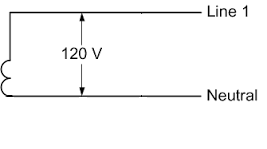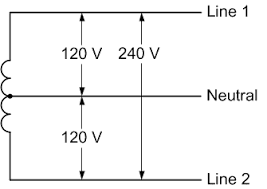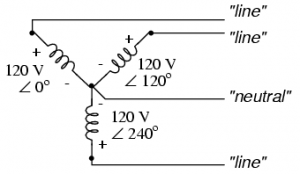## Breaking News

If you’re not electrically minded, think of 3 Phase vs Single Phase electric power as something easier to visualize like mechanical power. They’re very different, but both deliver power using pressure (force) and flow (speed). In both the power delivered is calculated by multiplying pressure (force) times flow (speed).
In mechanical power, many terms describe the pressure or force (Foot Pounds, Pounds per Square Inch, etc.) and many terms describe the speed or flow (Rotating Speed, Gallons per Minute, etc.). In electric power, one term describes the pressure or force (Voltage) and two terms describe the speed or flow (Current and Amperes).
In the earliest days Direct Current (DC), where the power flows in one direction like a water hose, was the standard for delivering electrical power.  Now Alternating Current (AC), where the power flow is constantly alternating direction, is the standard for delivering electrical power.

The standard for delivering electrical power changed from Direct Current (DC) to Alternating Current (AC) because Alternating Current (AC) delivers electrical power more efficiently over long distances.

• In the US, 60 Hertz (cycles per second) is the Alternating Current (AC) frequency.
• In some countries, 50 Hertz (cycles per second) is the Alternating Current (AC) frequency.?

What is 1 (Single) Phase Power ?If you’re not electrically minded, think of 1 (Single) phase power like a bicycle where only one leg (phase) is pushing on one pedal rotating around a crankshaft axis (neutral).

Mechanically, power is calculated as leg pressure (Foot Pounds) times speed (Rotating Speed).
Electrically, power is calculated as leg force (Voltage) times flow (Current).
Single Phase power is a two wire Alternating Current (AC) power circuit. Most people use it every day because it’s the most common household power circuit and powers their lights, TV, etc. Typically there’s one power wire and one neutral wire and power flows between the power wire (through the load) and the neutral wire.

In the US, 120V is the standard single phase voltage with one 120V power wire and one neutral wire.
In some countries, 230V is the standard single phase voltage with one 230V power wire and one neutral wire.
What is 2 (Dual / Split) Phase Power ?If you’re not electrically minded, think of 2 (Dual / Split) phase power like a bicycle where one leg (phase) can push on one pedal, or both legs (phases) can push on both pedals (180 degrees out of phase with one another) rotating around a crankshaft axis (neutral).

1. Mechanically, power is calculated as leg pressure (Foot Pounds) times speed (Rotating Speed).
2. Electrically, power is calculated as leg force (Voltage) times flow (Current).
Dual Phase or Split Phase power is also Single Phase because it’s a two wire Alternating Current (AC ) power circuit. In the US, this is the standard household power arrangement with two (Phase A, Phase B) 120V power wires (180 degrees out of phase with one another) like two bicycle pedals and one neutral wire. This arrangement is used in most US households because of its flexibility.

• Low power loads (lights, TV, etc.) powered using either either of (2) 120V power circuits
• High power loads (Water Heaters, AC Compressors) powered using (1) 240V power circuit

What is 3 (Three) Phase Power ?If you’re not electrically minded, think of 3 (Three) phase power like a three cylinder engine where three pistons (phases) located (120 degrees out of phase with one another) push rotating around a crankshaft axis (neutral).

1. Mechanically, I’m not sure how to calculate the power.
2. Electrically, power is calculated as cylinder force (Voltage) times flow (Current) times 1.732 (Square Root of 3).
Three Phase power is a three wire Alternating Current (AC) power circuit. Most US commercial buildings use a 3 Phase 4 Wire 208Y/120V power arrangement because of its power density and flexibility. Compared to single phase, a 3 phase power arrangement provides 1.732 (the square root of 3) times more power with the same current and provides (7) power circuits.

• Low power loads (Lights, etc.) powered using any of (3) 120V single phase power power circuits
• Medium power loads (Water Heaters, etc.) powered using any of (3) 208V single phase power circuits
• High power loads (HVAC Systems, etc.) powered using (1) 208V three phase power circuit
Most US industrial facilities use a 3 Phase 4 Wire 480Y/277V power arrangement because of its power density. Compared to 208V 3 Phase, 480V 3 Phase provides 2.3 (480 /208) times more power with the same current or 43% (208/480) less current with the same power. This yields additional benefits.

• Reduced construction costs with smaller electrical service, wiring, conduits, and electrical devices.
• Reduced energy costs will less energy lost as electrical current resistance (converted to heat).